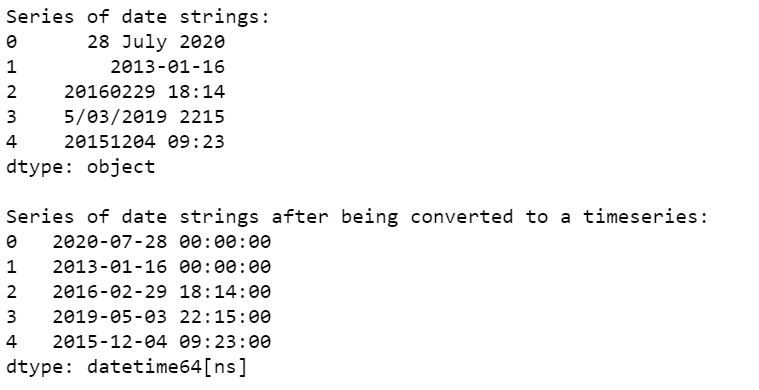Open In App
Related Articles
• Write an Interview Experience
• Pandas Exercises and Programs
• Different ways to create Pandas Dataframe

# Convert a series of date strings to a time series in Pandas Dataframe

During the analysis of a dataset, oftentimes it happens that the dates are not represented in proper type and are rather present as simple strings which makes it difficult to process them and perform standard date-time operations on them.

pandas.to_datetime() Function helps in converting a date string to a python date object. So, it can be utilized for converting a series of date strings to a time series.

Let’s see some examples:
Example 1:

## Python3

 `# import pandas library``import` `pandas as pd`` ` `# create a series of date strings``dt_series ``=` `pd.Series([``'28 July 2020'``, ``'16 January 2013'``,``                       ``'29 February 2016 18:14'``])`` ` `# display the series initially``print``(``"Series of date strings:"``)``print``(dt_series)`` ` `# display the series after being ``# converted to a time series``print``(``"\nSeries of date strings after"` `+` `       ``" being converted to a timeseries:"``)`` ` `print``(pd.to_datetime(dt_series))`

Output: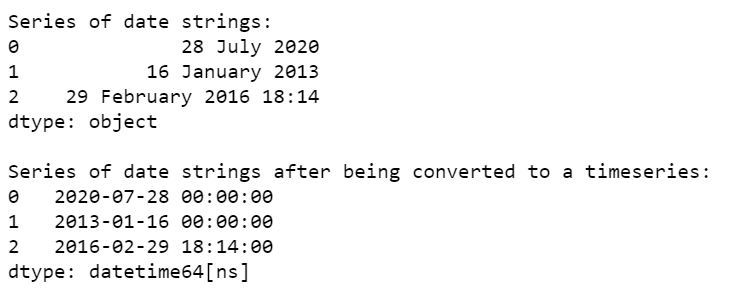Example 2:

## Python3

 `# import pandas library``import` `pandas as pd`` ` `# create a series of date strings``dt_series ``=` `pd.Series([``'2020/07/28'``, ``'2013/01/16'``,``                       ``'2016/02/29 18:14'``])`` ` `# display the series initially``print``(``"Series of date strings:"``)``print``(dt_series)`` ` `# display the series after being``# converted to a time series``print``(``"\nSeries of date strings after "` `+` `      ``"being converted to a timeseries:"``)`` ` `print``(pd.to_datetime(dt_series))`

Output: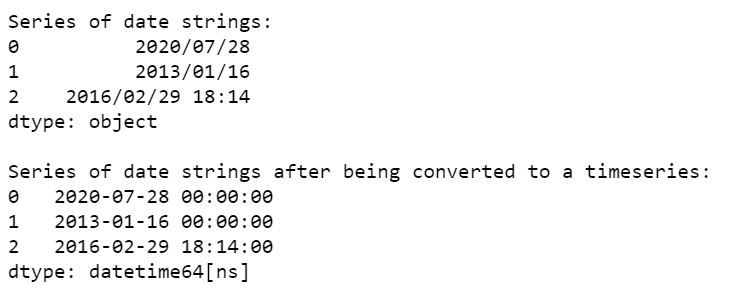Example 3:

## Python3

 `# import pandas library``import` `pandas as pd`` ` `# create a series of date strings``dt_series ``=` `pd.Series([``'2020-07-28'``, ``'2013-01-16'``, ``                       ``'2016-02-29 18:14'``])`` ` `# display the series initially``print``(``"Series of date strings:"``)``print``(dt_series)`` ` `# display the series after ``# being converted to a time series``print``(``"\nSeries of date strings after "` `+``      ``"being converted to a timeseries:"``)`` ` `print``(pd.to_datetime(dt_series))`

Output: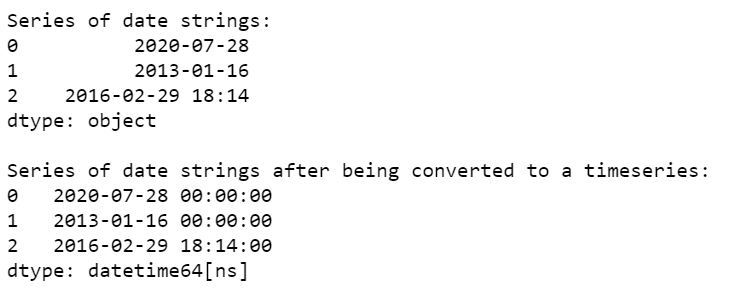Example 4:

## Python3

 `# import pandas library``import` `pandas as pd`` ` `# create a series of date strings``dt_series ``=` `pd.Series([``'28/07/2020'``, ``'01/16/2013'``, ``                       ``'29/02/2016 18:14'``])`` ` `# display the series initially``print``(``"Series of date strings:"``)``print``(dt_series)`` ` `# display the series after being``# converted to a time series``print``(``"\nSeries of date strings after "` `+``      ``"being converted to a timeseries:"``)`` ` `print``(pd.to_datetime(dt_series))`

Output: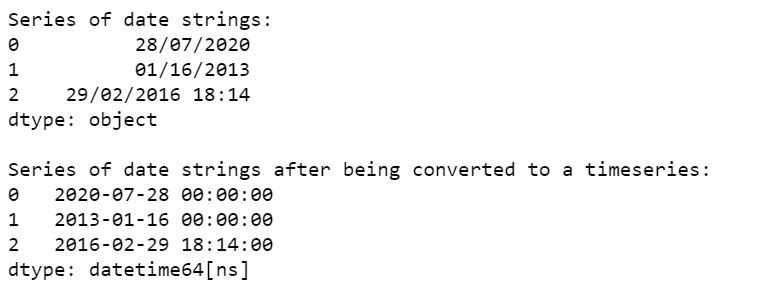Example 5:

## Python3

 `# import pandas library``import` `pandas as pd`` ` `# create a series of date strings``dt_series ``=` `pd.Series([``'20200728'``, ``'20130116'``, ``                       ``'20160229 181431'``])`` ` `# display the series initially``print``(``"Series of date strings:"``)``print``(dt_series)`` ` `# display the series after ``# being converted to a time series``print``(``"\nSeries of date strings after "` `+``      ``"being converted to a timeseries:"``)`` ` `print``(pd.to_datetime(dt_series))`

Output: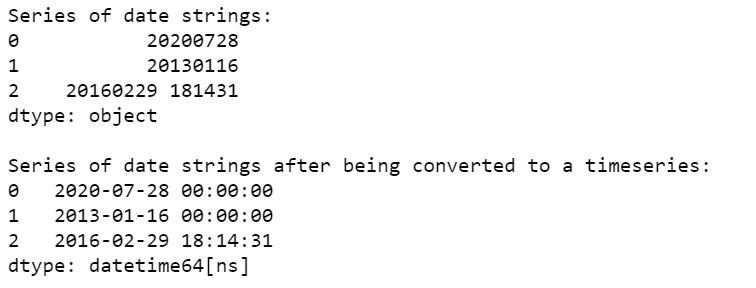Example 6:

## Python3

 `# import pandas library``import` `pandas as pd`` ` `# create a series of date strings``dt_series ``=` `pd.Series([``'28 July 2020'``, ``'2013-01-16'``,``                       ``'20160229 18:14'``, ``'5/03/2019 2215'``,``                       ``'20151204 09:23'``])`` ` `# display the series initially``print``(``"Series of date strings:"``)``print``(dt_series)`` ` `# display the series after ``# being converted to a time series``print``(``"\nSeries of date strings after "` `+` `      ``"being converted to a timeseries:"``)`` ` `print``(pd.to_datetime(dt_series))`

Output: